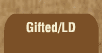Mathematics and Dyslexia

Tips for Learning the Times Tables

Tools for the Times Tables & Workbook

Home > Math > times tables practice > Sevens (all of them)

## The seven Times Tables (all of them)

The sevens are the penultimate group (that is, next to the last :)). You're really almost there :)

1. 7 x 10=
2. 7 x 5=
3. 3 x 7=
4. 7 x 9=
5. 7 x 7=
6. 7 x 1=
7. 6 x 7=
8. 7 x 8=
9. 2 x 7=
10. 8 x 7=
11. 0 x 7=
12. 7 x 4=
13. 7 x 6=
14. 7 x 4=
15. 7 x 8=